Science Research
Volume 4, Issue 6, December 2016, Pages: 169-173

Comparing System Performance of Optical OFDM Systems Using LMSTE, NLMSTE and DFTE

Department of Electrical Engineering, Faculty of Electrical Engineering and Computer Sciences, Islamic Azad University North Tehran Branch, Tehran, Iran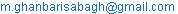Mohammad Ghanbarisabagh. Comparing System Performance of Optical OFDM Systems Using LMSTE, NLMSTE and DFTE. Science Research. Vol. 4, No. 6, 2016, pp. 169-173. doi: 10.11648/j.sr.20160406.14

Received: November 25, 2016; Accepted: January 17, 2017; Published: February 7, 2017

Abstract: The evaluation of Optical Orthogonal Frequency Division Multiplexing (OOFDM) using Least Mean Square Time-domain Equalizer (LMSTE), its normalized form (NLMSTE) and Decision Feedback Time-domain Equalizer (DFTE) to reduce Cyclic Prefix (CP) length over 1200 Km of Standard Single Mode Fiber (SSMF) is presented. All of these TEQs are used immediately after the fiber channel. They can cancel the residual Inter Symbol Interference (ISI) and Inter Carrier Interference (ICI) caused by both the Group Velocity Dispersion (GVD) and the CP length being shorter than the Channel Impulse Response (CIR). Using these TEQs allow the reduction in size of CP, and consequently leading to system performance improvement. Using DFTE can decrease the noise whereas using NLMSTE can solve the problem of choosing the gain of LMS algorithm to make it stable since LMS algorithm is sensitive to the scaling of its input.

Keywords: Optical Communications, OOFDM, Equalizer, SSMF

Contents

1. Introduction

Orthogonal Frequency Division Multiplexing (OFDM)  has been employed for fiber optic communication because it is an effective solution to ISI, when symbol period of each subcarrier is longer than the delay spread caused by GVD [2,3].

To eliminate the effect of ISI, OFDM system adds a CP to the waveform . If the CP length is sufficiently long, any time shift will not affect the received signal. Therefore, the subcarriers will remain orthogonal. But, the CP wastes transmission capacity, if its length is not optimized . When the CP length is longer than the CIR, ISI can be eliminated so that the effect of delay spread caused by GVD in long-distance SMF leads to frequency selective fading of individual sub-band channels. Luckily, this fading can easily be cancelled by using one-tap Frequency-domain Equalizer (FEQ) . However, this method will waste the energy within the CP, because the system leads to low energy efficiency. On the other hand, if the CP length is shorter than the CIR, energy wastage is reduced but the system performance will be limited by ISI .

Ghanbarisabagh et. al. earlier introduced the use of LMSTE, NLMSTE and DFTE in OOFDM for reducing CP size to 0.39% [7-11].

However, using DFTE can decrease the noise [7-10] whereas using NLMSTE can solve the problem of choosing the gain of LMS algorithm to make it stable since LMS algorithm is sensitive to the scaling of its input .

2. Comparison by System Performance

If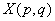denotes the frequency domain data symbol of the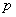-th sub-band of the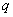-th OFDM symbol, then the transmitted OFDM symbol in discrete-time domain will be shown as:(1)

Where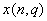is an N-point Inverse Fast Fourier Transform and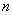is the time domain index of the OFDM sample.

Then the CP is added to the signal to avoid the ISI provided by the long- distance SMF Channel. If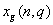and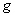denote the extended OFDM symbol and length of the CP, respectively, it can be written as: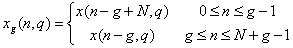(2)

After D/A conversion, the components are used to drive the electrical modulator. To provide an optical output power proportional to the electrical drive voltage, the optical modulator is assumed to be linearized. It has been shown that Mach-Zehnder modulators without linearization can be used in O-OFDM [4,10,11]. The modulator output is then coupled into the SMF channel. Receiver Gaussian Noise is then added to the channel output, so that the received samples,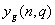is given by: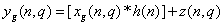(3)

where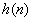and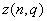are the discrete-time impulse response of the SMF Channel and Gaussian Noise in the time domain, respectively.

When the CP length is shorter than the CIR, the ISI will not be completely cancelled. The residual ISI reduces the performance of the system. Transformation of Equation (3) in frequency domain can be written by: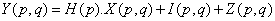(4)

where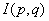is the residual ISI and ICI due to the CP length being shorter than the CIR. To overcome this residual ISI and ICI, a TEQ immediately after the channel and a 1-tap FEQ after FFT for each subcarrier are proposed. TEQ can provide multipath diversity. Furthermore, shorter CP length can reduce the energy wastage associated with the CP. Finally, the residual ISI can be cancelled by the CP and one-tap FEQ.

When the received samples,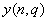is applied to the receiver, the LMS/NLMS/DFTEs estimates the channel information and determines the tap weights. This adaptive process is given by equations (5)-(8) for LMSTE and NLMSTE, respectively:(5)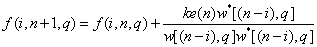(6)

where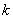,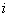,,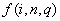and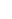are step size,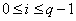, time domain index, tap weight and received training data of-th OFDM symbol respectively. The estimated erroris given by: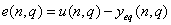(7)

where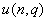is training data. Then, the TEs output can be obtained as: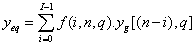(8)

Now, 1-tap FE can cancel the residual ISI for.

For DFTE, a Mean Square Error (MSE) criterion is used to decrease the noise. The MSE criterion can reduce the signal variance at the decision portion, so that the noise will decrease . If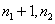and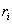are length of the feed-forward filter, length of the feed-back filter and DF-TEQ input, respectively, the DF-TEQ output can be written as: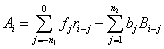(9)

whereand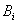are feed-forward filter coefficients, feed-back filter coefficients, decision device input and correct transmitted symbol, respectively.

The design of the DFTE is determined by the feed-forward and feed-back filter coefficients. The feed-forward filter coefficients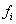, are given by: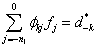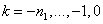(10)

where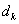are the CIR coefficients and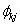can be written as: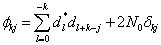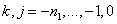(11)

where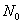is the variance of the noise and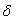is the delta function.

By using the feed-forward coefficients from (10) and (11), the feed-back filter coefficients are given by: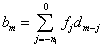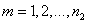(12)

3. Results and Discussions

To simulate the system, a direct-detection O-OFDM with double-sideband optical signal as shown in figure 1 is used. 512 sub-carriers with the sub-carrier spacing of 40 MHz achieving 20.48 Gb/s are modulated using a 4-QAM constellation The OFDM symbol duration is T=25 ns. 15 spans of 80 km standard SMF is used as transmission line. GVD characterizes the channel model with dispersion coefficient 17 Ps/(km-nm), wavelength 1.55 µm, fiber length of 1200 km and attenuation coefficient 0.2 dB/km. The photodiode noise is given by the contributions of shot noise and thermal noise which are independent random processes with approximately Gaussian statistics . After each span, amplifiers with a flat gain of 20 dB and a Noise Figure (NF) of 5 dB are used. Polarization Mode Dispersion (PMD) coefficient of the lab fibers is considered 0.07-ps/sqrt(km) resulting a mean Differential Group Delay (DGD) value of ~ 2.5 ps. To evaluate the system performance, the Bit Error Rate (BER) versus Optical Signal to Noise Ratio (OSNR) is plotted using MATLAB. The BER is measured over a 12.5 GHz noise bandwidth, which is equivalent to 0.1 nm at 1550 nm.

Figures 2-4 present the BER in terms of OSNR for the OOFDM receiver with using LMS//NLMS/DFTE for different size of CP while considering 6, 8, 10-tap LMS/NLMSTEs and 3/2, 4/2, 6/5 feedforward/feedback-tap DFTE, respectively. Additionally, the power penalties versus CP duration for LMS/NLMSTEs and NLMS/DFTEs are presented in Figs. 5 and 5, respectively.Figure 1. Direct Detection OFDM systems.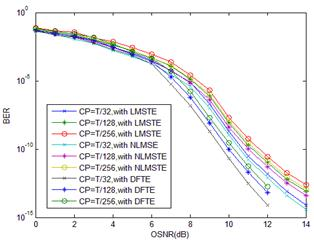Figure 2. BER versus OSNR using 6LMS/6NLMS/3-2 feedforward-feedback DFTEs.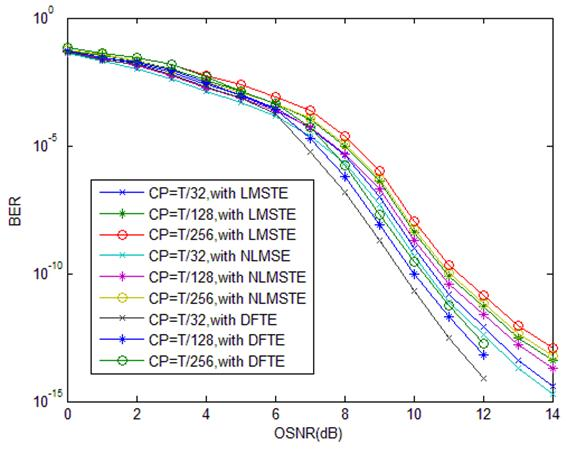Figure 3. BER versus OSNR using 8LMS/8NLMS/4-2 feedforward-feedback DFTEs.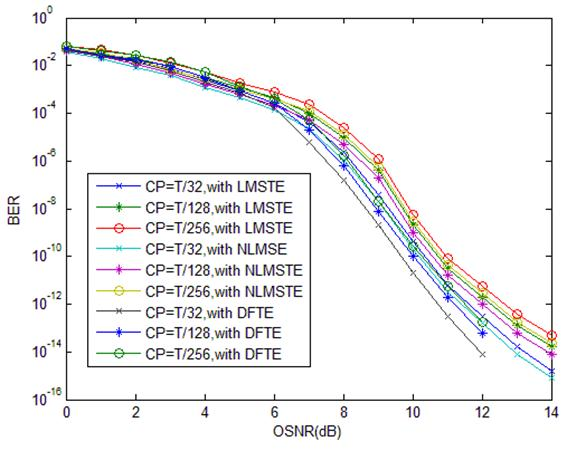Figure 4. BER versus OSNR using 10LMS/10NLMS/6-4 feedforward-feedback DFTEs.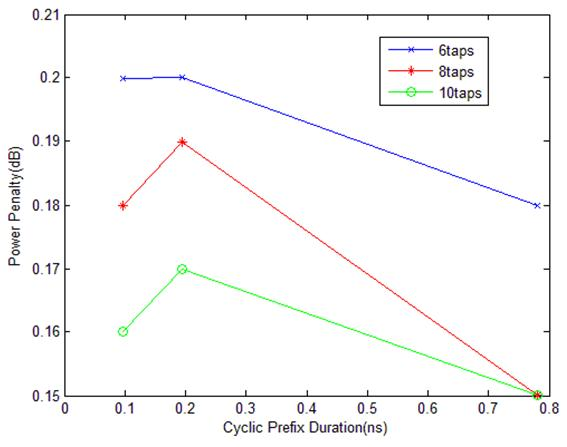Figure 5. Power penalty versus CP duration for LMS/NLMSTEs.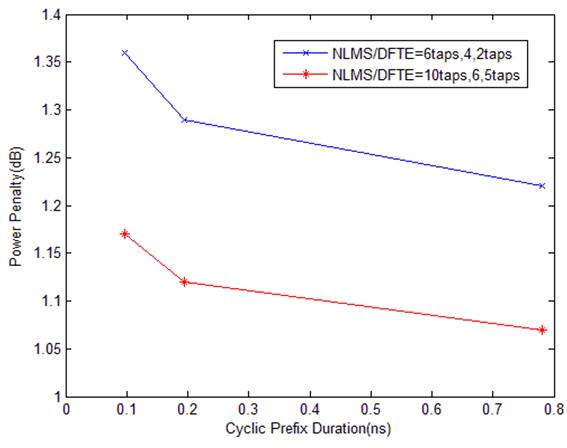Figure 6. Power penalty versus CP duration for NLMS/DFTEs.

In addition, the efficiency of the OFDM system can be defined as :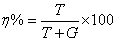(13)

where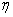,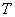and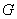are efficiency of the OFDM system, OFDM symbol duration and CP length, respectively. From (13), by using TEQs immediately after the channel, the calculation efficiency is 99.61% while considering CP=T/256.

4. Conclusion

In this paper, three TEQs are compared for Direct-Detection OOFDM system to cancel the residual ISI and ICI caused by both the GVD and the CP length being shorter than the CIR while considering 1200 km of SSMF. Based on simulation results, DFTE has better performance compared to the others.

References

1. J. G. Proakis and M. Salehi, Essentials of Communications Systems Engineering. Englewood Cliffs, NJ: Prentice Hall, 2005.
2. S. Jansen, I. Morita, T. Schenk, N. Takeda, and H. Tanaka, "Coherent optical 25.8-Gb/s OFDM transmission over 4160-km SSMF," J. Light w. Technol., vol. 26, no. 1, pp. 6–15, Jan. 2008.
3. D. J. F. Barros and J. M. Kahn, "Optimized dispersion compensation using orthogonal frequency-division multiplexing," J. Light w. Technol., vol. 26, no. 16, pp. 2889–2898, Aug. 2008.
4. A. J. Lowery and J. Armstrong, "Orthogonal frequency division multiplexing for dispersion compensation of long haul optical systems, "Opt. Express, vol. 14, no. 6, pp. 2079–2084, Mar. 2006.
5. H. Bolckei, p. Duhamel and R. Hleiss, " A subspace based approach to blind channel identification in pulse shaping OFDM/OQAM system," IEEE Trans. Signal Processing, vol.49, no.7, pp. 1594-1598, July 2001.
6. Richard Van Nee, OFDM for Wireless multimedia communication, Artech House, pp. 33-41, 2000.
7. M. Ghanbarisabagh, M. Y. Alias, H. A. Abdul-Rashid, "Cyclic prefix reduction for 20.48 Gb/s direct-detection optical OFDM transmission over 2560 km of SSMF," International Journal of Communication Systems, vol.24, no.11, pp. 1407-1417, November 2011.
8. M. Ghanbarisabagh, M. Y. Alias, H. A. Abdul-Rashid, "Comparison of LMS-TEQ and DF-TEQ to reduce cyclic prefix length in direct detection optical OFDM system," Optik-International Journal for Light and Electron Optics, vol.123, issue.11, pp. 941-946, June 2012.
9. M. Ghanbarisabagh, "Evaluation of O-OFDM communication systems using LMS-TEQ and DF-TEQ by efficiency and complexity," Optik-International Journal for Light and Electron Optics, vol.124, issue.17, pp. 2894-2896, September 2013.
10. M. Ghanbarisabagh, M. Y. Alias, H. A. Abdul-Rashid, "Performance Analysis of Least Mean Square Time-Domain Equalizer for 20.48 Gb/s Direct-Detection Optical OFDM Transmission Over 2560 km of SMF," 2009 IEEE 9th Malaysia International Conference on Communications (MICC 2009), 14-17, December 2009, Seri Pacific Hotel, Kuala Lumpur, Malaysia, pp.168-171.
11. M. Ghanbarisabagh, "Using NLMS-TEQ to Reduce CP Length for 20.48 Gb/s Direct-Detection Optical OFDM Transmission over 2400 Km of SSMF," Optik-International Journal for Light and Electron Optics, vol.124, issue.22, pp. 5874–5877, November 2013.
12. Edward A. Lee, David G. Messerschmitt, "Digital Communication," ( Kluwer Academic Publishers, Boston, 1988)..
13. Govind P. Agrawal, Fiber-Optic Communication Systems, in Wiley Series in Microwave and Optical Engineering, Kai Chang, Series Editor, 2002.
14. S. Armour, A. Nix, D. Bull, "Performance analysis of a pre-FFT equalizer design for DVB-T," IEEE Transactions on consumer Electronics 45(3), (1999).

 Contents 1. 2. 3. 4.
Article ToolsAbstractPDF(382K)